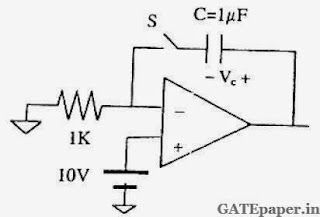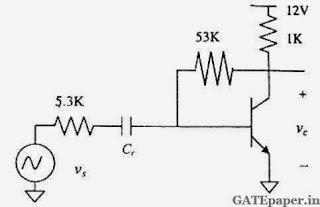### GATE 2006 ECE Video Solutions on Analog Circuits (Analog Electronics)

1. The input impedance(Zi) and output impedance (Zo) of an ideal Transconductance (voltage controlled current source) amplifier are
a. Zi = 0, Zo = 0
b. Zi = 0, Zo = ∞
c. Zi = ∞, Zo = 0
d. Zi = ∞, Zo = ∞

2. For the circuit shown in the following figure, the capacitor C is initially uncharged. At t = 0, the switch S is closed. The voltage VC across the capacitor at t = 1 ms is _____. Assume the OP – AMP is supplied with ± 15 volts.a. 0 volts
b. 6.3 volts
c. 9.45 volts
d. 10 volts

Common Data for Questions (3, 4 and 5):
In the transistor amplifier circuit shown in the figure below, the transistor has the following parameters: βDC = 60, VBE = 0.7 volts, hie -> ∞, hoe -> ∞. The capacitance CC can be assumed to be infinite.3. Under the DC conditions, the collector to emitter voltage drop is
a. 4.8 volts
b. 5.3 volts
c. 6.0 volts
d. 6.6 volts

4. If βDC is increased by 10%, the collector to emitter voltage drop
a. Increases by less than or equal to 10%
b. Decreases by less than or equal to 10%
c. Increases by more than 10%
d. Decreases by more than 10%

5. The small signal gain of the amplifier Vo/Vs is
a. – 10
b. – 5.3
c. + 5.3
d. + 10

A regulated power supply shown in the figure below has an unregulated input of 15 volts and generates a regulated output Vout. Use the component values shown in the figure.6. The power dissipation across the transistor Q1 shown in the figure is
a. 4.8 volts
b. 5.0 volts
c. 5.4 volts
d. 6.0 volts

7. If the unregulated voltage increases by 20%, then power dissipation across the transistor Q1
a. Increases by 20%
b. Increases by 50%
c. Remains unchanged
d. Decreases by 20%